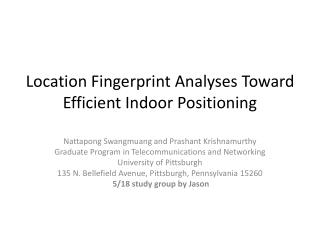DownloadDownload PresentationLocation Fingerprint Analyses Toward Efficient Indoor Positioning

# Location Fingerprint Analyses Toward Efficient Indoor Positioning

Download Presentation## Location Fingerprint Analyses Toward Efficient Indoor Positioning

- - - - - - - - - - - - - - - - - - - - - - - - - - - E N D - - - - - - - - - - - - - - - - - - - - - - - - - - -
##### Presentation Transcript

1. Location Fingerprint Analyses Toward Efficient Indoor Positioning NattapongSwangmuang and Prashant Krishnamurthy Graduate Program in Telecommunications and Networking University of Pittsburgh 135 N. Bellefield Avenue, Pittsburgh, Pennsylvania 15260 5/18 study group by Jason

2. Outline • Introduction & Related Work • Indoor Localization Fingerprint Model • Analytical Model for Probability Distribution • Performance Evaluation • Application to Offline phase

3. Introduction & Related Work • Traditional techniques: TOA: time of arrival, AOA: angle of arrival… • Modern techniques for indoor environment: Fingerprint, Bayesian modeling, Statistical learning…

4. Introduction & Related Work • Euclidean distance between some set < variation of RSS measured from those sets • The accuracy of deterministic approach or probabilistic approach has been reported to be similar • Employing proximity graphs for predicting performance of the system

5. Indoor Localization Fingerprint Model • WLAN based system • A square area with L*L=L^2 grids, using the mean of the RSS from N Aps in the area.

6. Indoor Localization Fingerprint Model • The sample vector is denoted as: R = [r1, r2, r3, ..., rN] • The random variables ri (in dBm) for all i are mutually independent. • The random variables ri(in dBm) are normally (or Gaussian) distributed. • The (sample) standard deviation of all the random variables ri is assumed to be identical and denoted by (in dB). • The mean of the random variable ri or E{ri} is denoted as ρi (in dBm).

7. Indoor Localization Fingerprint Model • The assumption that the RSS is normally distributed is acceptable. • When the APs are far from measured location and no direct LOS with, the distribution can be approximated by Gaussian. • R’ = [ρ1, ρ2, ρ3, ..., ρN] as fingerprint vector • Z=norm(R-R’), with central or non-central chi distribution.

8. Indoor Localization Fingerprint Model • PEP (pair-wise error prob.)and PCP(pair-wise correct prob.)=1-PEP Ri’ sdik’ Rk’

9. Indoor Localization Fingerprint Model • Ck = || Ri’ − Ri|| − || Rk’ − Ri|| is taken to calculate probability of correct decision: • With assumption of independence to simplify

10. Analytical Model for Probability Distribution • Voronoi Diagram is a set of fingerprints defined as a division of the space according to the nearest-neighbor rules.

11. Analytical Model for Probability Distribution • Finding neighbor set is relative close to the target location • Three proximity graph: • DG(Delaunay Graph): In the graph, there exists a Delaunay edge between two points u, v if they share the same Voronoi edge as boundary

12. Analytical Model for Probability Distribution • (GG)Gabriel Graph: a graph that contains a Gabriel edge between two points u, v – if a diametral circle from these two points contains no other point w. Satisfied: (ab)^2<(ac)^2+(bc)^2

13. Analytical Model for Probability Distribution • (RNG)Relative Neighbor Graph: a graph that contains an edge between two points u, v – if there is no other point w that is imultaneouslycloser to both points than they are to one another. Mathematically, sduv<=max[sduw, sdvw]. w w u v

14. Analytical Model for Probability Distribution • Given the Mobile Station is at grid i • Remove the effect from remote grids

15. Performance Evaluation We simulated 10,000 RSS samples from a given MS location and applied the nearest neighbor computation to estimate its location

16. Performance Evaluation

17. Performance Evaluation

18. Performance Evaluation

19. Performance Evaluation

20. Performance Evaluation

21. Performance Evaluation

22. Performance Evaluation

23. Application to Offline Phase • Strong RSSs(-60~-40dBm) have low standard deviation(1~2dB) at LOS areas • Week RSSs(-95~-85dBm) have high standard deviation(6~7dB) at NLOS areas • Eliminating unnecessary grids to save effort • Sparse grids on open and large areas • Still need trail-and-error to make sure ‘good’ fingerprints of grids.

24. Thanks and Q&A